# Profinite group

A topological group that is the projective limit of an inverse system of finite discrete groups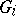,(whereis a pre-ordered directed set). The profinite groupis denoted by. As a subspace of the direct product, endowed with the compact topology (a neighbourhood base of the identity is given by the system of kernels of the projections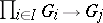), it is closed and hence compact.

### Examples.

1) Letbe the set of integers larger than zero with the natural order relation, and let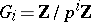. Suppose that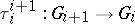is the natural epimorphism, and put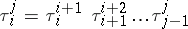for all. Then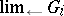is the (additive) group of the ring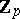of-adic integers.

2) Every compact analytic group over a-adic number field (e.g.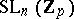) is profinite as a topological group.

3) Letbe an abstract group and letbe the family of its normal subgroups of finite index. Onone introduces the relation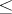, puttingif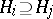. This relation turnsinto a pre-ordered directed set. Associate to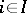the group, and to each pair,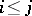, the natural homomorphism. One obtains the profinite group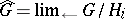, called the profinite group completion of. It is the separable completion of(cf. Separable completion of a ring) for the topology defined by the subgroups of finite index. The kernel of the natural homomorphism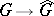is the intersection of all subgroups of finite index. In this construction one can consider, instead of the family of all normal subgroups of finite index, only those whose index is a fixed power of a prime number. The corresponding group is denoted by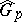, and is a pro--group.

4) Profinite groups naturally arise in Galois theory of (not necessarily finite) algebraic extensions of fields in the following way. Let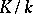be a Galois extension and suppose that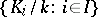is the family of all finite Galois extensions oflying in. Then, and one can introduce onthe relationby puttingif. The setthen becomes pre-ordered. Let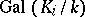be the Galois group of. To every pair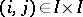,, one naturally associates the homomorphism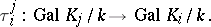The corresponding profinite group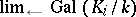is isomorphic to, thus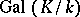can be considered as a profinite group. The systemforms ina neighbourhood base of the identity (cf. Galois topological group). This construction has a generalization in algebraic geometry in the definition of the fundamental group of a scheme.

A profinite group can be characterized as a compact totally-disconnected group (cf. Compact group), as well as a compact group that has a system of open normal subgroups forming a neighbourhood base of the identity. The cohomology theory of profinite groups (cf. Cohomology of groups; Galois cohomology) plays an important role in modern Galois theory.